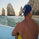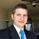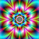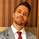# Ehlers Smoothed Stochastic & RSI with Roofing Filters27195 뷰
Roofing filters, first discussed by Mr.John Ehlers , act as a passband, filtering out unwanted noise from market data and accentuating turning points.

I have included 2 indicators with filters enabled. Both support double smoothing via options page. All the parameters are configurable.

Info on Roofing Filter and Ehlers Super Smoother:
----------------------------------------------------
The Ehlers' Roofing Filter is an expansion on Ehlers Super Smoother Filter, both being smoothing techniques based on analog filters. This filter aims at reducing noise in price data.

In Super Smoother Filter, regardless of the time frame used, all waves having cycles of less than 10 bars are considered noise (customizable via options page). The Roofing Filter uses this principle, however, it also creates a so-called "roof" by eliminating wave components having cycles greater than 48 bars which are perceived as "spectral dilation". Thus, the filter only passes those spectral components whose periods are between 10 and 48 bars. This technique noticeably reduces indicator lag and also helps assess turning points more accurately.

- Spectral dilation paper: http://www.mesasoftware.com/Seminars/Spe...

------------------------------------------------------
If you want to use RSI %B and Bandwidth, follow this guide to "Make mine" this chart and get access to the source:

For the complete list of my indicators, check this post:
오픈 소스 스크립트

진정한 TradingView의 정신에 따라, 이 스크립트의 작성자는 스크립트를 오픈소스로 게시했기에 거래자들이 이해하고 확인할 수 있습니다. 작가님께 건배! 스크립트를 무료로 사용할 수 있지만, 게시물에서 이 코드를 재사용하는 것은 하우스룰을 따릅니다. 당신은 스크립트를 차트에 사용하기 위해 그것을 즐겨찾기 할 수 있습니다.

차트에 이 스크립트를 사용하시겠습니까?
```//
// @author LazyBear
// List of all my indicators: https://www.tradingview.com/v/4IneGo8h/
//
study("Ehlers Smoothed Stochastic [LazyBear]", shorttitle="ESSTOCH_LB")
PI=3.14159265359
applyDoubleSmoothing=input(false, type=bool)
length = input (14, title="Stoch Length")
lengthMA=input (3, title="Stoch MA Length")
over_bought = input (.8)
over_sold = input (.2)
src=close
roofingBandUpper=input(48)
roofingBandLower=input(10)

a1 = exp(-PI * sqrt(2) / lower)
coeff2 = 2 * a1 * cos(sqrt(2) * PI / lower)
coeff3 = - pow(a1,2)
coeff1 = 1 - coeff2 - coeff3
filt = coeff1 * (price + nz(price)) / 2 + coeff2 * nz(filt) + coeff3 * nz(filt)
filt

EhlersRoofingFilter(price, smoothed, upper, lower) =>
alpha1 = (cos(sqrt(2) * PI / upper) + sin (sqrt(2) * PI / upper) - 1) / cos(sqrt(2) * PI / upper)
highpass = pow(1 - alpha1 / 2, 2) * (price - 2 * nz(price) + nz(price)) +
2 * (1 - alpha1) * nz(highpass) - pow(1 - alpha1, 2) * nz(highpass)
smoothed ? EhlersSuperSmootherFilter(highpass, lower) : highpass

EhlersStochastic(price, length, applyEhlerSmoothing, roofingBandUpper, roofingBandLower) =>
filt = EhlersRoofingFilter(price, applyEhlerSmoothing, roofingBandUpper, roofingBandLower)
highestP = highest(filt, length)
lowestP = lowest(filt, length)
iff ((highestP - lowestP) != 0, (filt - lowestP) / (highestP - lowestP),  0)

hline (over_bought)
hline (over_sold)
hline((over_bought+over_sold)/2)
plot(sma(stoch, lengthMA), color=red, linewidth=1)
plot(stoch, color=blue, linewidth=1)

```
List of my free indicators: http://bit.ly/1LQaPK8
List of my indicators at Appstore: http://blog.tradingview.com/?p=970

## 코멘트i cant seem to get the RSI to load on my charts and the script seems to only have the stoch ?
응답This indicator is in PineScipt V3 can any one convert it to V4.

i get error on these two lines: -

filt = coeff1 * (price + nz(price)) / 2 + coeff2 * nz(filt) + coeff3 * nz(filt)
filt

highpass = pow(1 - alpha1 / 2, 2) * (price - 2 * nz(price) + nz(price)) +
2 * (1 - alpha1) * nz(highpass) - pow(1 - alpha1, 2) * nz(highpass)
응답float filt = 0
filt := coeff1 * (price + nz(price)) / 2 + coeff2 * nz(filt) + coeff3 * nz(filt)
filt

float highpass = 0
highpass := pow(1 - alpha1 / 2, 2) * (price - 2 * nz(price) + nz(price)) +
2 * (1 - alpha1) * nz(highpass) - pow(1 - alpha1, 2) * nz(highpass)
응답@TradeShotsPro, Would you mind sharing this script in v4? I wasn't able to get it to work.
응답Выложите пожалуйста индикатор RSI with Roofing Filters, его нигде нет.
Спасибо!)
응답The second function

alpha1 = (cos(sqrt(2) * PI / upper) + sin (sqrt(2) * PI / upper) - 1) / cos(sqrt(2) * PI / upper)

should be

alpha1 = (cos(sqrt(2) * PI / (2 * upper)) + sin (sqrt(2) * PI / (2 * upper)) - 1) / cos(sqrt(2) * PI / (2 * upper))

Ehlers used 0.707 in his calculations for the spectral dilation ( = sqrt(2) / 2) so you are missing a '/2' somewhere in the multiplication chain of each trig function argument.
응답